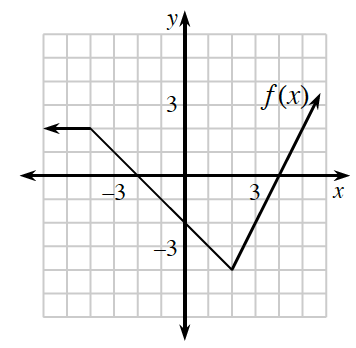### Home > PC3 > Chapter 2 > Lesson 2.2.3 > Problem2-86

2-86.Using the graph of $y=f(x)$ at right, sketch the following function transformations.

1. $y=f(2x)$

When $x=3$, the transformed function has a $y$-value of $f(6)$.
Therefore, what was $f(6)$ is now $f(3)$.

2. $y=f\left(\frac{1}{2}x\right)$

When $x=4$, the transformed function has a $y$-value of $f(2)$.

3. $y=f(-x)$

Think about the relationship between the location of '$x$' and '$-x$'.

It reflects the graph across the $y$-axis.